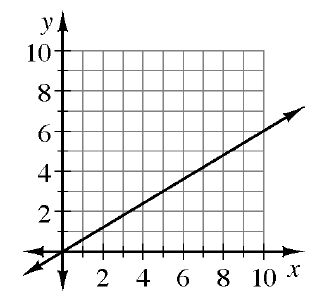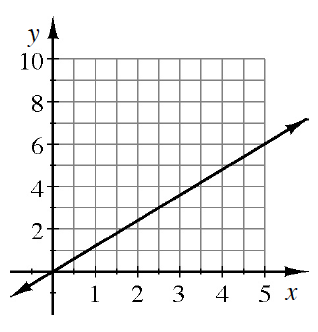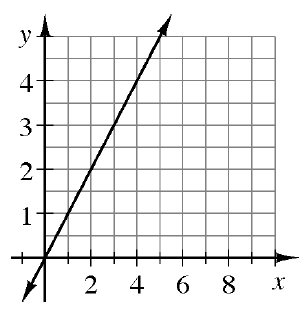### Home > CC3MN > Chapter 7 > Lesson 7.2.3 > Problem7-50

7-50.

Find the slope of each line below.

1.Slope can be defined as the change in $y$ over the change in $x$.

Pick two whole-number points on the line.

The coordinates for two points on the line are (10, 6) and
(5, 3). Now count the change in $y$ and the change in $x$.

$\frac{3}{5}$

1.Pick two points on the line.

The coordinates of two points are (5, 6) and (0, 0).
Find the slope. See part (a) for help.

1.See part (a).